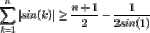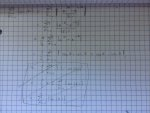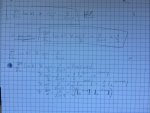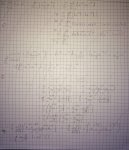# Prove sum [k=1,n] |sin(k)| >= (n+1)/2 - 1/(2 sin(1))

##### New member
Hello, I'm trying to prove this but I'm not really succeeding.I've tried different things :I don't know if I have to prove it starting from the definition of sinus, or if I have to do a mathematical induction, starting from n to prove it for n+1.
I'd really appreciate some help, thank you.

#### stapel

##### Super Moderator
Staff member
I'm trying to prove this but I'm not really succeeding:

. . . . .$$\displaystyle \displaystyle \sum_{k=1}^{n}\, \big| \,\sin(k)\, \big| \, \geq\, \dfrac{n\, +\, 1}{2}\, -\, \dfrac{1}{2\, \sin(1)}$$

I've tried different things...
In your first image, I think you did something circular with the following identity:

. . . . .$$\displaystyle \sin(x)\, =\, \dfrac{e^{ix}\, -\, e^{-ix}}{2i}$$

I can't figure out what you're doing in the second image...? You seem to be assuming what you need to prove, and then bringing the above identity in near the end...?

The text in the third image is small and then is all scratched out, so I can't say much on that.

I don't know if I have to prove it starting from the definition of sinus, or if I have to do a mathematical induction, starting from n to prove it for n+1.
What kind of class are you in? (Graduate school real-analysis? Calculus? etc) What topics have recently been studied? This information may help us figure out how you're probably expected to proceed.

Thank you!